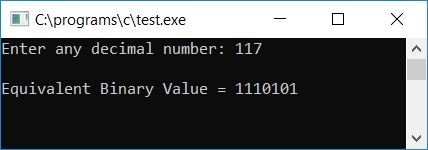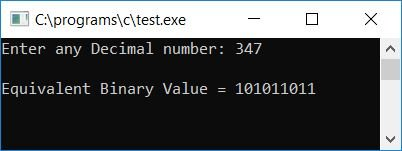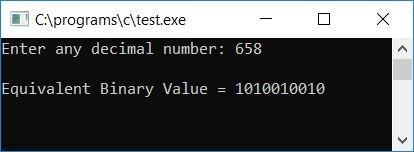# C Program to Convert Decimal to Binary

In this article, we will learn how to create a program in C that converts any given number (in a decimal number) entered by the user at run-time into its equivalent value in a binary number. At last, we have also created a program that uses user-defined functions to do the same job.

But before going through the program, if you are not aware of

• Decimal Number
• Binary Number
• Decimal to Binary Conversion

Then, refer to the step-by-step process of converting decimal to binary. Now let's move on to the program.

## Decimal to binary in C

To convert a decimal number to a binary number in C programming, you have to ask the user to enter the number (in the decimal number system) to convert it into a binary number and then display the equivalent value in binary as an output.

```#include<stdio.h>
#include<conio.h>
int main()
{
int decnum, binnum, i=0;
printf("Enter any decimal number: ");
scanf("%d", &decnum);
while(decnum!=0)
{
binnum[i] = decnum%2;
i++;
decnum = decnum/2;
}
printf("\nEquivalent Binary Value = ");
for(i=(i-1); i>=0; i--)
printf("%d", binnum[i]);
getch();
return 0;
}```

As the above program was written in the Code::Blocks IDE, here is the sample run after a successful build and run. This is the first snapshot of the sample run:Now supply any number (in decimal), say 117, and press the ENTER key to see its equivalent binary value as shown in the second snapshot of the sample run given here:#### Program Explained

• Receive any decimal number from the user at program runtime.
• Create a while loop that runs until the value of the decimal number (decnum) becomes 0.
• Let's suppose that the user has entered 117 as input.
• Therefore, at the first run of the while loop, decnum!=0 or 117!=0 evaluates to true, and therefore the program flow goes inside the loop.
• And decnum%2 or 117%2 or 1 (because if we divide 117 by 2, we'll get 1 as a remainder) is set to binnum[i] or binnum. This is because i was set to 0 at the beginning of the program, so at the first time through the loop, i still has a value of 0.
• Now, the value of the variable "i" is increased by 1 and becomes 1, and decnum/2 or 117/2 or 58 is set to decnum as the initial value.
• Again, program flow returns to the condition of the while loop.
• As a result, on the second iteration of the while loop, decnum!=0 or 58!=0 evaluates to true, and program flow returns to the loop.
• And decnum%2 or 58%2 or 0 (because when 58 is divided by 2, it doesn't leave a remainder this time, so the remainder is 0) is set to binnum.
• The value of i gets incremented and becomes 2. And finally, decnum/2 or 58/2 or 29 gets initialized to decnum.
• Now, at the third run of the while loop, decnum!=0 or 29!=0 evaluates to true, and now, for the third time, the program flow goes inside the loop and does the similar steps as described in the above steps until and unless the value of decnum becomes 0. Here the value of decnum at the first, second, third, fourth, fifth, sixth, seventh, and eighth runs will be 117, 58, 29, 14, 7, 3, 1, and 0.
• After storing equivalent binary value of given decimal number. We have to print the value of binary digit in reverse order.
• That is, create a for loop that runs from i-1. Here we have subtracted the value of i from 1 because, at the last run of the while loop, the value of i gets incremented and decnum/2 gets initialized to decnum. And when the value of decnum becomes 0 (the last run of the loop), the program flow does not go inside the loop again after checking the condition decnum!=0 or 0!=0 (that evaluates to false), but we have already incremented the value of i. Therefore, we have to subtract it from 1 to delete its extra index.
• Therefore, in the for loop, we have started the loop from i-1, which runs until it is greater than or equal to 0.
• Print the value of binnum[i] one by one.
• In this way, we have the equivalent binary value of the given decimal number, which is printed on the output console.

### Decimal to Binary in C without using an array

The question is: write a program in C that converts a decimal number to binary without using an array. The answer to this question is given below:

```#include<stdio.h>
#include<conio.h>
int main()
{
int decnum, binnum=0, mul=1, rem;
printf("Enter any decimal number: ");
scanf("%d", &decnum);
while(decnum>0)
{
rem = decnum%2;
binnum = binnum+(rem*mul);
mul = mul*10;
decnum = decnum/2;
}
printf("\nEquivalent Binary Value = %d", binnum);
getch();
return 0;
}```

This program produces the same output as the previous program.

### Decimal to Binary in C without a Modulus Operator

If you want to create the same program but without using the modulus operator (%), then

• divide the number by 2, and initialize the quotient value to any variable, say temp.
• Now multiply the quotient by 2 and initialize its multiplication result to another variable, say chk.
• and then check whether the value of chk is equal to the original value (the current value of decnum, the dividend) or not.
• There is no remainder if it is equal; otherwise, there is a remainder if it is not equal.
• and if the remainder does not leave, then we have to initialize 0 to binnum[i] (i holds 0 at the first run).
• Otherwise, if there is any remainder, we must initialize 1 to the binnum array's initial index (0th index), increment the index of the array, and continue as shown in the program below.

The question is, "Write a program in C that converts decimal to binary without using the modulus operator." The answer to this question is:

```#include<stdio.h>
#include<conio.h>
int main()
{
int decnum, binnum, i=0, temp, chk;
printf("Enter any Decimal number: ");
scanf("%d", &decnum);
while(decnum!=0)
{
temp = decnum/2;
chk = temp*2;
if(chk==decnum)
binnum[i] = 0;
else
binnum[i] = 1;
i++;
decnum = temp;
}
printf("\nEquivalent Binary Value = ");
for(i=(i-1); i>=0; i--)
printf("%d", binnum[i]);
getch();
return 0;
}```

Here is the final snapshot of the sample run:### Decimal to Binary in C using a User-Defined Function

Now let's create a user-defined function named DecToBin() that receives one argument (the decimal number) to convert and stores its equivalent binary value one by one in the bin[] array as shown in the program given below. The question is, "Write a program in C that converts a decimal number to a binary number using a user-defined function." The answer to this question is:

```#include<stdio.h>
#include<conio.h>
void DecToBin(int dec);
int bin;
static int i;
int main()
{
int decnum;
printf("Enter any decimal number: ");
scanf("%d", &decnum);
DecToBin(decnum);
printf("\nEquivalent Binary Value = ");
for(i=(i-1); i>=0; i--)
printf("%d", bin[i]);
getch();
return 0;
}
void DecToBin(int dec)
{
while(dec!=0)
{
bin[i] = dec%2;
i++;
dec = dec/2;
}
}```

Here is the final snapshot of the sample run:Here we have declared the array bin[] and variable i outside the function main(), as after declaring both outside the main() function (at the start of the program), the array (bin[]) and variable (i) both stay known and can be used throughout the program. The value of i is declared as a static variable. Because a static variable holds its previous value. And one more thing about static variables: if you do not initialize any value to a static variable initially, then it holds 0 as its initial value.

#### The same program in different languages

C Quiz

« Previous Program Next Program »